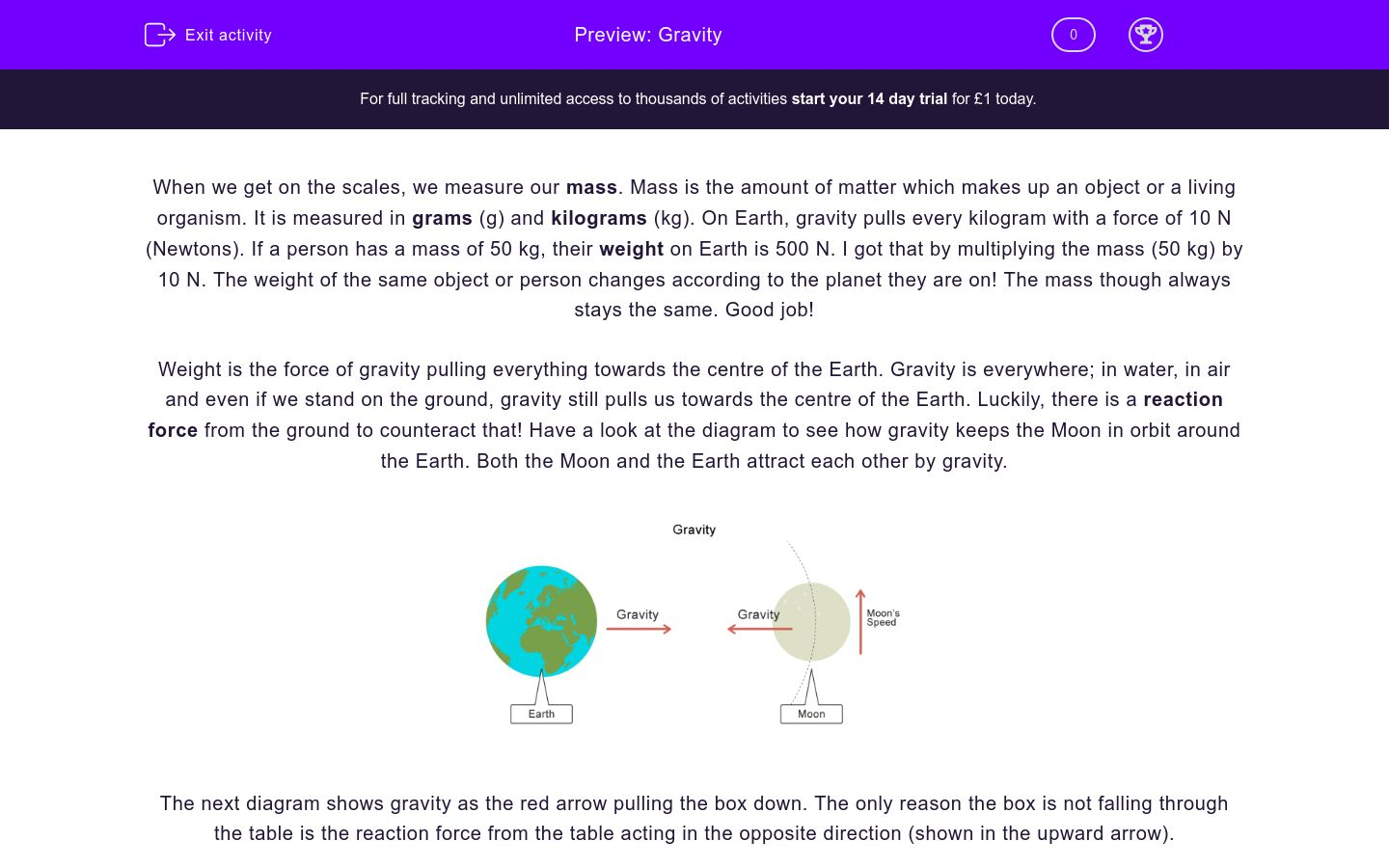# Gravity

In this worksheet, students will learn about gravity in our life and how mass is different to weight.Key stage:  KS 3

Curriculum topic:   Physics: Space Physics

Curriculum subtopic:   Gravity Forces

Difficulty level:### QUESTION 1 of 10

When we get on the scales, we measure our mass. Mass is the amount of matter which makes up an object or a living organism. It is measured in grams (g) and kilograms (kg). On Earth, gravity pulls every kilogram with a force of 10 N (Newtons). If a person has a mass of 50 kg, their weight on Earth is 500 N. I got that by multiplying the mass (50 kg) by 10 N. The weight of the same object or person changes according to the planet they are on! The mass though always stays the same. Good job!

Weight is the force of gravity pulling everything towards the centre of the Earth. Gravity is everywhere; in water, in air and even if we stand on the ground, gravity still pulls us towards the centre of the Earth. Luckily, there is a reaction force from the ground to counteract that! Have a look at the diagram to see how gravity keeps the Moon in orbit around the Earth. Both the Moon and the Earth attract each other by gravity.The next diagram shows gravity as the red arrow pulling the box down. The only reason the box is not falling through the table is the reaction force from the table acting in the opposite direction (shown in the upward arrow).What is mass?

gravity pulling

the amount of matter things are made of

the reaction force from the ground

What is weight?

the amount of matter things are made of

someone's weight on the Moon

the force of gravity pulling everything

What is the unit for measuring mass? Two answers are correct.

g

G

kg

N

What is the unit for measuring weight?

g

kg

N

If an object has a mass of 4 kg, what is its weight on Earth?

40 kg

40 N

2.5 N

Is this statement true or false?

There is no gravity in the sea.

true

false

Where does gravity pull us to?

towards the centre of the Earth

upwards

downwards

What is the force that prevents gravity making everything fall?

friction

air resistance

reaction force

What keeps the Moon in orbit around the Earth?

What is the weight of an object with a mass of 7 kg on Earth?

700 N

7 N

70 N

• Question 1

What is mass?

the amount of matter things are made of
EDDIE SAYS
Mass is the amount of matter things are made of.
• Question 2

What is weight?

the force of gravity pulling everything
EDDIE SAYS
Weight is the force of gravity pulling everything.
• Question 3

What is the unit for measuring mass? Two answers are correct.

g
kg
EDDIE SAYS
Mass is measure in g and kg.
• Question 4

What is the unit for measuring weight?

N
EDDIE SAYS
Weight is a force, so it is measured in N as all forces.
• Question 5

If an object has a mass of 4 kg, what is its weight on Earth?

40 N
EDDIE SAYS
The weight is 4 x 10 = 40 N
• Question 6

Is this statement true or false?

There is no gravity in the sea.

false
EDDIE SAYS
This is false. Gravity is everywhere!
• Question 7

Where does gravity pull us to?

towards the centre of the Earth
EDDIE SAYS
Gravity on Earth pulls us towards the centre of the Earth.
• Question 8

What is the force that prevents gravity making everything fall?

reaction force
EDDIE SAYS
Reaction force prevents gravity making everything fall.
• Question 9

What keeps the Moon in orbit around the Earth?

gravity
Gravity
EDDIE SAYS
Gravity keeps the Moon in orbit around the Earth.
• Question 10

What is the weight of an object with a mass of 7 kg on Earth?

70 N
EDDIE SAYS
7 x 10 = 70 N
---- OR ----

Sign up for a £1 trial so you can track and measure your child's progress on this activity.

### What is EdPlace?

We're your National Curriculum aligned online education content provider helping each child succeed in English, maths and science from year 1 to GCSE. With an EdPlace account you’ll be able to track and measure progress, helping each child achieve their best. We build confidence and attainment by personalising each child’s learning at a level that suits them.

Get started